# International Mathematical Talent Search

IMTS Rounds Index: 1û9 10 11 12 13 14 15 16 17 18 19 20

##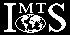Solutions and Comments Round 10

### Problem 1/10

Find x2 + y2 + z2 if x, y, and z are positive integers such that

7x2 û 3y2 + 4z2 = 8   and   16x2 û 7y2 + 9z2 = û3 .

### Solution 1:

Refer to 7x2 û 3y2 + 4z2 = 8 as (1) and 16x2 û 7y2 + 9z2 = û3 as (2). Then 16ò(1) û 7ò(2) produces the equation y2 + z2 = 149, while 7ò(1) û 3ò(2) produces the equation x2 + z2 = 65. In the set of positive integers, the first of these yields the solutions (y, z) = (10, 7), (7, 10), while the second one yields (x, z) = (1, 8), (8, 1), (7, 4), (4, 7). Comparing these, we find that z = 7 and that x = 4 and y = 19. Upon checking that these values indeed satisfy the original equations, we can compute that x2 + y2 + z2 = 165.

### Solution 2:

The system of equations,
 7x2 û 3y2 + 4z2 = 8, 16x2 û 7y2 + 9z2 = û3, x2 + y2 + z2 = S,
is linear in x2, y2, z2. Upon solving it (using Gaussian elimination or some other method), we find that x2 = S û 149, y2 = S û 65, z2 = 214 û S. From these, since x2, y2, z2 are positive, it follows that 149 < S < 214. In this range, S û 65 is a perfect square only for S = 165, 186, 209. For these values we get 214 û S = 49, 28, 5 . The only one of these producing a perfect square is S = 165. Since 165 û 149 is also a perfect square it follows that the desired answer is indeed 165.

### Comments:

When we have two equations in three unknowns, we know that in general, we will not find a unique solution based on the equations alone. It is only the further condition that x, y and z are positive integers, that allows us to produce a unique solution.

Alternately, one finds that 9ò(1) û 4ò(2) produces y2 û z2 = 84. This equation may also be solved in integers by factoring both sides, but the previous solutions are more straightforward.

### Problem 2/10

Deduce from the simple estimate, 1 < Í3 < 2, that 6 < 3Í3 < 7 .

### Solution 1:

Í3 < 2 Þ Í3 + 1 < 3 Þ 2Í3 + 1 < 23 = 8 < 9 = 32 Þ 2 < (32)1/(Í3 + 1) = 3Í3 û 1 Þ 6 < 3Í3 Þ 18 < 3Í3 + 1 Þ 42 < 3Í3 + 1 Þ 4Í3 û 1 = 42/(Í3 + 1) < 3 Þ 4Í3 < 12 < 49/4 Þ 4Í3 + 1 < 72 Þ 4 < 72/(Í3 + 1) = 7Í3 û 1 Þ 28 < 7Í3 Þ 27 < 7Í3 Þ 3Í3 < 7 .

### Solution 2:

1 < Í3 < 2 Þ 0 < Í3 û 1 < 1 Þ 0 < (Í3 û 1)2 < 1 Þ 0 < Í3 û (3/2) < 1/2 Þ 0 < (Í3 û (3/2))2 < 1/4 Þ 5/3 < Í3 < 7/4 Þ 35/3 < 3Í3 < 37/4 Þ 6 < 3Í3 < 7 since 63 = 216 < 243 = 35 Þ 6 < 35/3 and 37 = 2187 < 2401 = 74 Þ 37/4 < 7 .

### Comments:

Alternately, 5/3 < Í3 follows from 3(2 û Í3) < (2 + Í3)(2 û Í3) = l, and Í3 < 7/4 follows from 1 = (2 + Í3)(2 û Í3) < 4(2 û Í3). One can also observe that 1 < Í3 < 2 Þ 1 < 2 Þ 2/9 < 4/9 and l/16 < 2/16 Þ 25/9 < 27/9 and 48/16 < 49/16 Þ 5/3 < Í3 and Í3 < 7/4 upon taking square roots. These same bounds on Í3 can also be obtained by applying the inequalities 1 < Í3 and Í3 < 2 on the right side of the identity

 Í3 = 1 + 1ùûùùùûù1 + 1 / (1+Í3)

Since neither the hypothesis nor the conclusion involved tight bounds, it would have been a violation of the ôspirit of the problemö to obtain and utilize sharper approximations than the ones used above.

This problem, as well as the next one, were originally posed by Mr. F. David Hammer some years ago. Mr. Hammer is presently an industrial mathematician/computer analyst; some years ago, during his academic career, he prepared the problems for the excellent Stockton State Mathematics Contest.

### Problem 3/10

For each positive integer n, n ³ 2, determine a function

fn(x) = an + bn x + cn |x û dn| ,

where an, bn, cn, dn depend only on n, such that

fn(k) = k + 1   for   k = 1, 2, ... , n û 1   and   fn(n) = 1 .

### Solution 1:

For x ú dn, fn(x) = an + cndn + (bn û cn)x, while for x ³ dn, fn(x) = an û cndn + (bn + cn)x . Of course, these functions coincide when x = dn . From the fact that for 1 ú x ú n û 1, fn(x) = x + 1, we know that for x ú n û 1, the slope of the function, bn û cn = 1, and the y-intercept, an + cndn = 1 . Since the slope of the function must become negative at some point before x = n, in order for fn(n) = 1, we know that n û 1 ú dn < n . If we choose dn = n û 1, then we have the slope of the second part of the function, bn + cn = 1 û n, so we can solve for bn and cn in terms of n to obtain bn = 1 û n/2 and cn = ûn/2 , and so an = 1 + n(n û 1) / 2 .

### Solution 2:

More generally, recognizing that any dn in the interval [n û 1, n) may be chosen, we find that the slope of the second part of the function is ûdn / (n û dn) . Now solving for bn and cn yields bn = 1 û n / (2(n û dn)) and cn = ûn / (2(n û dn)) . Thus an = 1 + ndn / (2(n û dn)) .

### Solution 3:

One must recognize that the flip-flop behavior of fn must be caused by dn ; i. e., one must choose dn so that the sign of k û dn changes when k = n . To this end, dn must satisfy n û 1 < dn < n . Then, for k = 1, 2, 3, ..., n, we are led to the following equations:

an + bn + cn(dn û 1 ) = 2 ,
an + 2bn + cn(dn û 2) = 3 ,
:
:
an + (n û 1)bn + cn(dn û (n û 1)) = n ,
an + nbn + cn(n û dn) = 1 .

From the first two of these we find that cn = bn û 1, while the first and last equations ù upon substituting for cn ù yield an = n/2 + 1 û nbn . Hence, upon substituting these values for an and cn, from the first equation we find that bn = 1 û n / (2(n û dn)) , which in turn leads to an = 1 + ndn / (2(n û dn)) and cn = ûn / (2(n û dn)) where dn = n û e with 0 < e < 1 . For example, if dn = n û 1/2, then (an, bn, cn, dn) = ((2n2 û n + 2)/2, 1 û n, ûn, n û 1/2)) .

### Problem 4/10

A bag contains 1993 red balls and 1993 black balls. We remove two balls at a time repeatedly and
1. discard them if they are of the same color,
2. discard the black ball and return to the bag the red ball if they are different colors.
What is the probability that this process will terminate with one red ball in the bag?

### Solution:

First observe that since at least one ball is removed during each turn, the process will eventually terminate with 0 or 1 ball in the bag. Since black balls can be removed 1 or 2 at a time, the number of black balls will eventually be zero. However, since red balls are removed 2 at a time and since we start with an odd number of red balls, the number of red balls in the bag at any given time will be odd. Therefore, the probability that the process terminates with 1 red ball remaining in the bag is l.

### Comments:

This problem was created by Daniel Macks, a former USAMTS participant. Dan is presently an undergraduate at Brown University.

### Problem 5/10

Let P be a point on the circumcircle of DABC, distinct from A, B, and C. Suppose BP meets AC at X, and CP meets AB at Y. Let Q be the point of intersection of the circumcircles of DABC and DAXY, with Q A. Prove that PQ bisects the segment XY. (The various points of intersection may occur on the extensions of the segments.)

### Solution:

We shall complete the proof in the case of an arrangement of points A, B, C, and P on the circumcircle of DABC satisfying the conditions of the problem and such that both points X and Y lie outside of that circle. Such an arrangement is shown in the figure below; the case where one of X or Y lies inside the circumcircle of DABC may be treated in a similar fashion.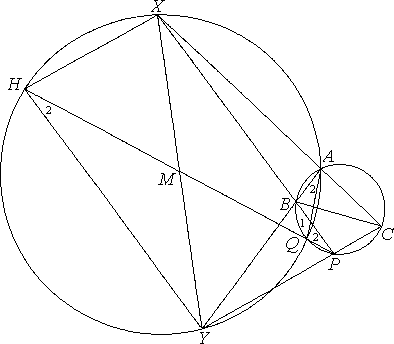Referring to the figure on the right, note that PQ has been extended to meet the circumcircle of DAXY at poÝnt H. The reader should recall that the measure of an angle inscribed in a circle equals one-half the measure of its intercepted arc. From this result, it follows that two angles inscribed in a circle and intercepting a common arc are equal and also that, in a cyclic quadrilateral, opposite angles are supplementary. We shall be applying these results to four cyclic quadrilaterals of our figure: ACPQ and ABQP (both inscribed in the circumcircle of DABC) and AQHX and HAQY (both inscribed in the circumcircle of DAXY).

Since ðACP and ðAQP are opposite angles of ACPQ, they are supplementary. So

ðACP equals ðAQH .     (1)

Since ðAQH and ðHXA are opposite angles of AQHX,

ðAQH and ðHXA are supplementary .     (2)

From (1) and (2), it follows that ðACP and ðHXA are supplementary. Thus segments HX and YC must be parallel.

Now ðBAQ and ðBPQ are both inscribed in the circumcircle of DABC and both intercept arc BQ. So ðBAQ equals ðBPQ. A similar argument in the circumcircle of DAXY reveals that ðYAQ equals ðYHQ. Since ðYAQ coincides with ðBAQ, ðBPQ equals ðYHQ. Thus segments XP and HY must be parallel.

It follows that XHYP is a parallelogram, and its diagonals must bisect each other. Thus, XY is bisected by PH, which is the extension PQ.

### Comments:

This problem is a generalization of Problem 17055 in the ôMathematical Questions and Solutionsö of the Educational Times [21(1912):41]. It was proposed by Dr. Stanley Rabinowitz, whose Index to Mathematical Problems, 1980û1984 is highly recommended to all problemists. The solution given above (as well as the figure) are also due to him.
IMTS Rounds Index: 1û9 10 11 12 13 14 15 16 17 18 19 20

##Solutions and Comments Round 11

### Problem 1/11

Express 19/94 in the form 1/m + 1/n , where m and n are positive integers.

### Solution 1:

First observe that since 19/94 < 1/4 , both m and n are greater than 4. To obtain an upper bound on them, note that 19/94 = 1/m + 1/n = (m + n) / mn ; thus 94(m + n) = 19mn . Since 47 is a prime divisor of 94, it follows that either m or n is divisible by 47. Without loss of generality, assume that 47 divides n. Thus 1/n ú 1/47 , and 1/m = 19/94 û 1/n > 19/94 û 1/47 = 17/94 > 1/6 . It follows that 4 < m < 6 , and hence m can only be 5. When m = 5 , we see that 1/n = 19/94 û 1/5 = 1/470 , and the unique answer is

 19ù94 = 1ù5 + 1ùù470 .

### Solution 2:

The equation 19/94 = (m + n) / mn can be written in the form (19m û 94)(19n û 94) = 942. Let a = 19m û 94 and b = 19n û 94. Then m = (a + 94) / 19 , n = (b + 94) / 19 , and it follows that a + 94 0 (mod 19) and b + 94 0 (mod 19) . Thus a 1 (mod 19) and b 1 (mod 19) . Since the only positive integers congruent to 1 modulo 19 and less than 94 are 1, 20, 39, 58 and 77, and since of these only 1 divides 942, it follows that either a or b must be 1. With a = 1 we obtain (m, n) = (5, 470) as the unique solution.

### Solution 3:

From the equivalent equation, (19m û 94)(19n û 94) = 942 = 8836 , we find that 19m û 94 must be a factor of 8836. Since the factors of 8836 are F = 1, 2, 4, 47, 94, 188, 2209, 4418, 8836, it follows that m = (F + 94) / 19 . Without loss of generality, F ú 94. We try all possible values of F and get the following possible values of m:
 F : 1 2 4 47 94 m : 5 96ù19 98ù19 141ùù19 188ùù19

Since m must be an integer, it follows that m = 5, which yields n = 470, as before.

### Solution 4:

Assume, without loss of generality, that m < n , and hence that 1/m > 1/n . Upon observing that 1/5 < 19/94 < 1/4 and that 1/m < 19/94 = 1/m + 1/n < 2/m , it follows that 1/m < 1/4 and 2/m > 1/5 . Thus the only possible values for m are: 5, 6, 7, 8, 9. Of these, only m = 5 yields an integer value, 470, for n.

### Solution 5:

Again, assume that 1/m > 1/n . Since 1/5 < 19/94 < 1/4 , it follows that 5 ú m . Thus 19/94 û 1/m ³ 19/94 û 1/5 = 1/470 . Hence, 5 ú m < n ú 470 . We may then do a computer search in this range, and find that m = 5, n = 470 is the unique solution.

### Comments:

More generally, the problem may be recognized as a special case of the identity
 mùùùmk û 1 = 1ùk + 1ùùùùk (mk û 1) , with m = 19 and k = 5 .
Even more generally, the problem belongs to the topic of ôEgyptian Fractionsö. It can be proved that for given a and b, there are positive integers m and n such that a/b = 1/m + 1/n if and only if b has distinct relatively prime divisors s and t for which s + t 0 (mod a) .

One procedure for expressing a/b as the sum of ôunit fractionsö (i. e., fractions of the form 1/n) is to let n1 = Úb/a¨ , the smallest integer greater than b/a (This is the ôceiling functionö of b/a). Then n1 û b/a < 1, and hence an1 û b < a. Moreover, a/b û 1/n1 = (an1 û b) / bn1 . Repeat the process with (an1 û b) / bn1 in place of a/b. Since an1 û b < a , this process must terminate in a finite number, say k steps. When ank û b = 1 we are done. This process is known as the ôGreedy Algorithmö and the proof that it terminates relies on the ôWell-Ordering Propertyö of the natural numbers: Any nonempty subset of the natural numbers has a smallest element.

### Problem 2/11

Let n be a positive integer greater than 5. Show that at most eight members of the set {n + 1, n + 2, ... , n + 30} can be primes.

### Solution 1:

Observe that all members of the set Sn = {n + 1, n + 2, ... , n +30} can be expressed in the form 30N + i , where N is a non-negative integer (which may not be the same for all members of Sn) and i ranges from 0 to 29. Note that 30N + i is a multiple of 2 for i = 0, 2, 4, ... , 28; it is a multiple of 3 for i = 0, 3, 6, ... ,27; and it is a multiple of 5 for i = 0, 5, 10, ... , 25. Hence, for n > 5, the only values of i for which 30N + i could be prime are 1, 7, 11, 13, 17, 19, 23, and 29. Therefore, for n > 5, there can be at most eight primes in Sn .

### Solution 2:

By the Inclusion-Exclusion Principle, there are
 Ù 30ù2 ¹ + Ù 30ù3 ¹ + Ù 30ù5 ¹ û Ù 30ùù2ò3 ¹ û Ù 30ùù2ò5 ¹ û Ù 30ùù3ò5 ¹ + Ù 30ùù2ò3ò5 ¹ = 22
multiples of 2, 3, and 5 among any 30 consecutive positive integers. Hence if 2, 3, and 5 are not in the set Sn = {n + 1, n + 2, ... , n + 30}, then there are at least 22 composite numbers in Sn . Therefore, for n > 5, there are at most 30 û 22 = 8 primes in Sn .

### Solution 3:

For n > 5 , 15 of the 30 members of Sn = {n + 1, n + 2, ... , n + 30} are multiples of 2. Of the remaining 15 members of Sn , 5 are multiples of 3. And of the remaining 10 numbers, 2 must be multiples of 5. Therefore, 15 + 15 + 2 members of Sn must be multiples of 2, 3, or 5, and hence canÆt be primes. That leaves at most 30 û 22 = 8 primes in Sn .

### Solution 4:

Let Sn = {n + 1, n + 2, ... , n + 30}, and observe that for each d {1, 3, 7, 9} there must be three members of Sn, whose last digit is d. When n > 5 , these are the only candidates for primes since all numbers ending in 5 are multiples of 5, and those ending in an even digit are multiples of 2. Next observe that for each k = 0, l, 2, ... , one of the three numbers, k, k + 10, or k + 20 must be a multiple of 3. Hence, for each d {1, 3, 7, 9}, at most two of the members of Sn which end in d can be primes. Therefore, Sn can contain at most 4ò2 = 8 primes.

### Solution 5:

For k > 5, let Sk = {k + 1, k + 2, ... , k + 30}, and let s(k) be the statement ôthere are 22 multiples of 2, 3, or 5 in Sk.ö After verifying that s(6) is true, assume that s(k) is true for k = n . Then if n + 1 is a multiple of 2, 3, or 5, then so is n + 31 = (n + 1) + 30, and hence Sn+1 must also have 22 multiples of 2, 3, or 5. (We lose one, n + 1, as we go from Sn to Sn+1 , but gain one, n + 31, in its place.) On the other hand, if n + 1 is not a multiple of 2, 3, or 5, then all 22 multiples of 2, 3, and 5 must be in the subset {n + 2, n + 3, ... , n + 30} of Sn . Since this subset is also a subset of Sn+1 , it follows that s(n + 1) must be true. This completes the induction, and it follows that s(k) is true for all k > 5.

### Comments:

It is interesting to note that in addition to S6 , one finds that S1273 also contains eight primes: 1277, 1279, 1283, 1289, 1291, 1297, 1301, 1303. Is it true that for every positive integer N there is a positive integer n such that n > N and Sn contains eight primes? The original and the present problem may also be generalized to longer sequences of consecutive integers.

### Problem 3/11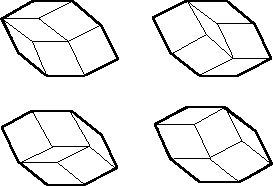A convex 2n-gon is said to be ôrhombicö if all of its sides are of unit length and if its opposite sides are parallel. As exemplified on the right (for the case of n = 4), a rhombic 2n-gon can be dissected into rhombi of sides 1 in several different ways.

For what value of n can a rhombic 2n-gon be dissected into 666 rhombi?

### Solution 1:

We will first prove by induction that a convex rhombic 2n-gon can be decomposed into
 ( n2 ) = n (n û 1)ùùùù2 unit rhombi.
The base case for n = 2 is true, since a rhombic 4-gon is already a single rhombus, and
 ( 22 ) = 2 (2 û 1)ùùùù2 = 1 .
Next we prove that any rhombic 2n-gon can be decomposed into n û 1 unit rhombi and a 2(n û 1)-gon. Choose one side of the rhombic 2n-gon, and orient the 2n-gon so that the chosen side is at the top. Denote the right-hand endpoint of the chosen side as vertex V1 and number the successive vertices clockwise. From each vertex, beginning with vertex V2 , draw a unit line segment, parallel to the chosen side, extending into the interior of the 2n-gon, until vertex Vnû1 is reached. No line segment can be drawn from vertex Vn , since, by the definition of the rhombic 2n-gon, the side between vertices Vn and Vn+1 is parallel to the chosen side. Now complete the n û 1 unit rhombi along the right side of the 2n-gon. Since, for each rhombus, the top and bottom sides are of unit length and parallel and the right sides are of unit length, the left sides are parallel to the right sides, and are also of unit length. Thus the left sides are also parallel to the opposite sides of the original 2n-gon. Thus the un-subdivided polygon remaining is a rhombic 2(n û 1)-gon, and it is convex because we have merely translated its right side one unit to the left. By the induction hypothesis, this rhombic 2(n û 1)-gon can be decomposed into
 ( n û 12 ) = (n û 1)(n û 2)ùùùùù2
unit rhombi, so the rhombic 2n-gon can be decomposed into
 (n û 1)(n û 2)ùùùùù2 + n û 1 = (n û 1)(n û 2) + 2n û 2ùùùùùùùùù2 = n2 û 3n + 2 + 2n û 2ùùùùùùùùù2 = n2 û nùùù2 = n (n û 1)ùùùù2 = ( n2 )
unit rhombi. Therefore, the problem is simplified to solving the quadratic equation
 ( n2 ) = 666 .
It can be written as (n + 36)(n û 37) = 0 , whose only positive solution is n = 37 . ThatÆs the solution to our problem.

### Solution 2:

First observe that a rhombic 2n-gon is completely determined by the sequence of its n interior angles, beginning at a chosen vertex. Such a rhombic 2n-gon can be constructed using unit rhombi (thus proving that it can be decomposed into unit rhombi) using the following algorithm:

 Initialization: Choose a side of the rhombic 2n-gon and place it at the top, and number the interior angles that determine the rhombic 2n-gon sequentially (clockwise) from a1 to an , starting at the vertex that is the right endpoint of the chosen side. Number the chosen side s1 and number the sides sequentially (clockwise) also. Now draw a horizontal unit line segment (call it e1 and, at an angle the same measure as angle a1 , draw another unit line segment emanating from the right-hand endpoint of e1 . Call this new segment e2 , and complete the rhombus determined by these two sides. Set i = 2 . Loop: While i < n , orient the 2i-gon so that the edge ei is horizontal at the top, and draw a unit segment from the right-hand endpoint of edge ei at an angle having the same measure as angle ai ; call this segment ei+1 . Draw a unit segment parallel to ei+1 emanating from the left-hand endpoint of each unlabeled edge, and complete the i unit rhombi determined by these segments and the rhombic 2i-gon. Replace i by i + 1 .

Since each iteration produces i more rhombi, the total number of rhombi produced in constructing a rhombic 2n-gon will be

 1 (from the initialization) + 2 + 3 + ... + (n û 1) = n (n û 1)ùùùù2 = ( n2 ) .
As in Solution 1, the equation
 ( n2 ) = 666
yields n = 37.

### Solution 3:

Construct an inner æringÆ of rhombi as follows. Complete the rhombus defined two adjacent sides. Now complete all possible rhombi around the perimeter of the 2n-gon. The result is 1 + (n û 1) + (n û 1) = 2n û 3 unit rhombi (see Solution 1) and a (2n û 4)-gon. This, together with the special cases for n = 2 and n = 3, establishes the recursion. Letting R(n) be the number of rhombi into which a rhombic 2n-gon can be decomposed, we find that R(2) = 1, R(3) = 3, and R(n) = R(n û 2) + 2n û 3 for n 4. This can be solved or simply evaluated until it is determined that R(37) = 666 .

### Comments:

While in Solution 1, one first had to guess the number of unit rhombi resulting from the dissection of a rhombic 2n-gon, Solutions 2 and 3 were constructive. In the problem (as well as in the solutions), we have assumed that the 2n-gon is convex; was that assumption necessary? What happens if the 2n-gon is concave? One may also inquire about the number of distinct dissections of a rhombic 2n-gon; that problem seems to be more difficult.

### Problem 4/11

Prove that if three of the interior angle bisectors of a quadrilateral intersect at one point, then all four of them must intersect at that point.

### Solution 1:

Let ABCD be a quadrilateral, and assume that

ðOAB = ðOAD , ðOBA = ðOBC , and ðOCB = ðOCD ,

where O is the intersection point of the angle bisectors at A, B, and C . Let E, F, G, and H be the feet of the perpendiculars from O to the sides of the quadrilateral, as shown in the figure below. Then DAEO and DAOH are congruent, since ðOAE = ðOAH, ðOEA = ðOHA, and |OA| = |OA| . Therefore, |OE| = |OH| . One can similarly show that |OE| = |OF| and |OF| = |OG| . Therefore, one may conclude that |OH| = |OG| .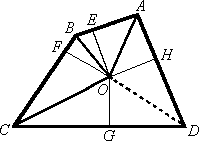To conclude the solution, we must still show that ðODG = ðODH . This can be done in several ways. For instance, if we construct a circle with radius |OG| and center O , then since GD and DH are tangent to the circle, the line OD will bisect the angle at D .

Alternately, one can argue that the right triangles DOGD and DODH are congruent, as they have the same hypotenuse and equal legs.

### Solution 2:

Consider the figure shown below, and apply the Law of Sines to DABO, DBCO, DCDO, and DDAO. Then the following equations will result:
 sin aùù|BO| = sin bùù|AO| , sin bùù|CO| = sin gùù|BO| , sin gùù|DO| = sin xùù|CO| ,   and sin yùù|AO| = sin aùù|DO| .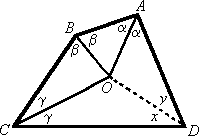Setting the product of the left hand sides equal to the product of the right hand sides of the above equations produces (after cancellations) the equation sin x = sin y . Since x + y 180░, it follows that x = y . Therefore, DO bisects ðADC, as claimed.

### Comments:

More generally, one can show that if n û 1 of the interior angle bisectors of an n-gon intersect in one point, then all n of them must intersect in that point. One may also note that if three of the angle bisectors of a quadrilateral intersect in one point, then one can inscribe a circle in the quadrilateral. Does this property generalize to n-gons?

### Problem 5/11

Let f(x) = x4 + 17x3 + 80x2 + 203x + 125 . Find the polynomial, g(x), of smallest degree for which f(3 ▒ Í3) = g(3 ▒ Í3) and f(5 ▒ Í5) = g(5 ▒ Í5) .

### Solution 1:

Let g(x) be any such polynomial and consider the polynomial h(x) = f(x) û g(x) . Then h(3 ▒ Í3) = 0 and h(5 ▒ Í5) = 0; i. e., h(x) is divisible by

(x û 3 û Í3)(x û 3 + Í5)(x û 5 û Í5)(x û 5 + Í5)
= (x2 û 6x + 6)(x2 û 10x + 20) = x4 û 16x3 + 86x2 û 180x + 120 .

Thus, there is a polynomial a(z) such that

f(x) û g(x) = h(z) = a(x)(x4 û 16x3 + 86x2 û 180x + 120) ,

and therefore

 g(x) = f(x) û a(x)(x4 + 16x3 + 86x2 û 180x + 120) = (x + 17x3 + 80x2 + 203x + 125) û a(x)(x4 û 16x3 + 86x2 û 180x + 120) .

Now g(x) will be of degree less than 4 if and only if a(x) 1 . In that case g(x) = 33x3 û 6x2 + 383x + 5, and this is the desired polynomial.

### Solution 2:

We are seeking the polynomial g(x) of least degree for which the graph passes through the four points (3 ▒ Í3, f(3 ▒ Í3)) = (3 ▒ Í3, 2864 ▒ 1337Í3) and (5 ▒ Í5, f(5 ▒ Í5)) = (5 ▒ Í5, 8340 ▒ 2963Í5) . Such a polynomial of degree less than or equal to 3 exists and, letting it be g(x) = ax3 + bx2 + cx + d , we need to require that the four linear equations

a(3 ▒ Í3)3 + b(3 ▒ Í3)2 + c(3 ▒ Í3) + d = 2864 ▒ 1337Í3
a(5 ▒ Í5)3 + b(5 ▒ Í5)2 + c(5 ▒ Í5) + d = 8340 ▒ 2963Í5

be satisfied. This system has the unique solution a = 33, b = û6, c = 383, and d = 5. Therefore, the desired polynomial is of degree 3 (otherwise we would have a = 0, for degree 2, or a = b = 0, for degree 1, or a = b = c = 0, for degree 0), and it is g(x) = 33x3 û 6x2 û 383x + 5 .

### Comments:

Clearly, the first solution is the less computational, and hence it is preferable. For the second solution, it is advisable to utilize a computer algebra system (CAS), rather than allowing for possible roundings, and hence loss of accuracy.
IMTS Rounds Index: 1û9 10 11 12 13 14 15 16 17 18 19 20

##Problems Round 13

### Problem 1/13

Milo is a student at Mindbender High. After every test, he figures his cumulative average, which he always rounds to the nearest whole percent. (So 85.49 would round down to 85, but 85.50 would round up to 86.) Today he has two tests. First he got 75 in French, which dropped his average by 1 point. Then he got 83 in History, which lowered his average another 2 points. What is his average now?

### Problem 2/13

Erin is devising a game and wants to select four denominations out of the available denominations \$1, \$2, \$3, \$5, \$10, \$20, \$25, and \$50 for the play money. How should he choose them so that every value from \$1 to \$120 can be obtained by using at most seven bills?

### Problem 3/13

For which positive integers d is it possible to color the integers with red and blue so that no two red points are a distance d apart, and no two blue points are a distance 1 apart?

### Problem 4/13

Prove that there are infinitely many ordered triples of positive integers (x, y, z) such that x3 + y5 = z7.

### Problem 5/13

Armed with just a compass ù no straightedge ù draw two circles that intersect at right angles; that is, construct overlapping circles in the same plane, having perpendicular tangents at the two points where they meet.
IMTS Rounds Index: 1û9 10 11 12 13 14 15 16 17 18 19 20

##Solutions and Comments Round 14

### Problem 1/14

Let a, b, c, d be positive numbers such that a2 + b2 + (a û b)2 = c2 + d2 + (c û d)2. Prove that a4 + b4 + (a û b)4 = c4 + d4 + (c û d)4.

### Solution 1:

Consider the square of the left side of the original equation:

 (a2 + b2 + (a û b)2)2 = (a4 + b4 + (a û b)4) + 2a2b2 + 2a2(a û b)2 + 2b2(a û b)2 = (a4 + b4 + (a û b)4) + 2a2b2 + 2(a2 + b2)(a û b)2 = (a4 + b4 + (a û b)4) + 2a2b2 + ((a + b)2 + (a û b)2)(a û b)2 = (a4 + b4 + (a û b)4) + 2a2b2 + (a2 û b2)2 + (a û b)4 = 2(a4 + b4 + (a û b)4) .

We can do the same thing for the expression with c and d. Thus, by squaring the original equation and dividing by two, the result follows.

### Solution 2:

By expanding (a û b)2 in the original equation, we see that the left hand side is equivalent to 2(a2 + b2 û ab) or equivalently 2((a û b)2 + ab) . We now divide the equation by 2 and square it to obtain ((a û b)2 + ab)2 = ((c û d)2 + cd)2 . From here, we will manipulate the expression on the left hand side of the equation:

 ((a û b)2 + ab)2 = (a û b)4 + 2ab(a û b)2 + a2b2 = (a û b)4 + 2a3b + 2ab3 û 3a2b2 = (a û b)4 + (ûa4 + 4a3b û 6a2b2 + 4ab3 û b4 + a4 + b4) / 2 = (a4 + b4 + (a û b)4) / 2 .

Thus, (a4 + b4 + (a û b)4) / 2 = (c4 + d4 + (c û d)4) / 2 , or equivalently, a4 + b4 + (a û b)4 = c4 + d4 + (c û d)4 .

### Solution 3:

Let x = (a û b)2, y = a2 + b2, u = (c û d)2, and v = c2 + d2. Note that y û x = 2ab which implies that (y û x)2 = 4a2b2 , so that
 a4 + b4 + (a û b)4 = y2 û 2a2b2 + x2 = x2 + y2 û 1ù2 (y û x)2 = 1ù2 (x + y)2.
Doing the analogous manipulations for c and d we get c4 + d4 + (c û d)4 = (u + v)2 / 2 . Thus, we seek to show that (x + y)2 / 2 = (u + v)2 / 2 given that x + y = u + v . At this point, the result is obvious.

### Problem 2/14

The price tags on three items in a store are as follows:

 \$ 0.75 \$ 2.00 \$ 5.50

Notice that the sum of these three prices is \$8.25, and that the product of these three numbers is also 8.25 . Identify four prices whose sum is \$8.25 and whose product is also 8.25 .

### Solution:

We will show that the four prices are \$0.50, \$2.00, \$2.75 and \$3.00 . To this end, first note that if x is any one of the prices (in dollars) then by the ArithmeticûGeometric Mean Inequality we have (8.25 û x) / 3 ³ (8.25 / x)(1/3) for any of the other prices. This implies .473 < x < 4.6069 . Thus, all prices must be between \$0.48 and \$4.60, inclusive.

Let a, b, c, and d be the prices (in cents). We wish to find a, b, c, d so that

a + b + c + d = 825,
abcd = 8.25 ò 1004 = 26 ò 3 ò 58 ò 11,
48 £ a, b, c, d < 460.

Suppose, without loss of generality, that a is not divisible by 5. Then 58 divides bcd. Since each of these integers is less than 54 = 625, none is divisible by 54. Therefore, 5 divides each of b, c and d. But this would imply that 5 does not divide a + b + c + d = 825, a contradiction. Therefore all the integers are divisible by 5.

Suppose, without loss of generality, that a is not divisible by 25. Then 57 divides bcd. If 25 divides each of b, c, and d then 25 does not divide a + b + c + d = 825, a contradiction. Thus, without loss of generality, b is also not divisible by 25, while 125 divides both c and d. Thus c and d must be one of the numbers {125, 250, 375}. We may check each of these values for c, d and solve for a and b. Doing this, we find that:

 if {c, d} = {125, 125}, then a, b ╗ 460.29, 114.71; if {c, d} = {125, 250}, then a, b ╗ 380.65, 69.35; if {c, d} = {125, 375}, then a, b ╗ 256.35, 68.65; if {c, d} = {250, 250}, then a, b ╗ 277.4, 47.6; if {c, d} = {250, 375}, then a, b ╗ 134.6, 65.4; if {c, d} = {375, 375}, contradicting that 9 is not a divisor of abcd.

Therefore, 25 must divide each of a, b, c, d. Let A = a / 25, B = b / 25, C = c / 25 and D = d / 25. We wish to find integers A, B, C, D satisfying:

A + B + C + D = 33,
ABCD = 26 ò 3 ò 11,
2 £ A, B, C, D £ 18.

Now, the only multiple of 11 between 2 and 18 is 11. Therefore, without loss of generafity, we may set A = 11. Thus we have

B + C + D = 22,
BCD = 26 ò 3
2 £ B, C, D £ 18.

Thus {B, C, D} {2, 3, 4, 6, 8, 12, 16}. Without loss of generality, 3 divides B, thus B = 3, 6 or 12. Checking each case, we find

 B = 3, {C, D} = {(19 ▒ Í105) / 2}, B = 6, {C, D} = {8 ▒ 4Í2}, B = 12, {C, D} = {2, 8}.

Since only the last case yields integer solutions, the four prices must be \$0.50, \$2.00, \$2.75 and \$3.00 .

### Problem 3/14

In a group of eight mathematicians, each of them finds that there are exactly three others with whom he/she has a common area of interest. Is it possible to pair them off in such a manner that in each of the four pairs, the two mathematicians paired together have no common area of interest?

### Solution 1:

Line up the mathematicians in the following order: Any mathematician may be first; in the second spot, put one of the four with whom the first shares no interest; in the third spot, put one of those who shares an interest with number 2; and for number 4, we choose a mathematician who shares no area of interest with number 3 (since there must be at least three left meeting this qualification). Then there will be at least one person sharing an interest with number 4, since at worst 4 could share interests with 1 and 2, so let that person be number 5. Since at most number 5 could have nothing in common with l, 2 and 3, we know that there is another mathematician with whom 5 shares no interest, and we will put that person sixth. Number 1 can be paired with 2, 3 with 4, and 5 with 6. If the remaining mathematicians (7 and 8) can be paired, then we are done. If they cannot, then we note that each of 7 and 8 can be legally paired with four of 1 though 6, so there must be a pair among (1, 2), (3, 4) and (5, 6) such that one of the pair can be paired with 7 and the other with 8.

### Solution 2:

Consider a graph having 8 vertices and each vertex having 3 edges incident to it. This means there are 12 edges in the graph. Remove any pair of nonadjacent vertices, along with the (6) edges incident to them. There are now 6 vertices and 6 edges. If all vertices have degree 2 (2 edges incident to each vertex), then 2 vertices and 4 edges can be removed by removing another pair of nonadjacent vertices. Otherwise, at least one vertex must have three edges incident to it. Remove a vertex of degree three and a nonadjacent vertex. This results in removing at least four edges. Now there are 4 vertices and at most 2 edges. If one vertex has degree 2, remove it and the nonadjacent vertex, leaving a legal pair. Otherwise the edges have no common endpoint, and removing any nonadjacent pair will remove both edges, leaving a legal pair.

### Solution 3:

Consider the complementary graph, whose 8 vertices represent the mathematicians, and an edge is drawn between two vertices if the corresponding mathematicians do not share an interest. Each vertex in this graph has degree 4. DiracÆs Theorem, which is also a corollary of OreÆs Theorem, states that a graph on n vertices, each vertex having degree greater than or equal to (n û 1) / 2, contains a Hamiltonian cycle (a cycle that begins at some vertex and passes through each other vertex exactly once before returning to the starting vertex). We can create the desired pairing by choosing alternate edges from a Hamiltonian cycle.

### Comments: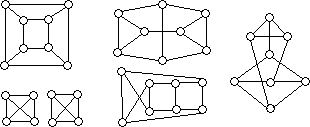The following graphs illustrate some of the possible combinations of shared interests among the eight mathematicians. In each of these cases, one can demonstrate that a pairing of the desired nature is possible. An alternate solution to the problem can be obtained by proving that the illustrations below exhaust all possibilities (do they?), and then by designating a pairing in each case.

### Problem 4/14

For positive integers a and b, define a ~ b to mean that ab + 1 is the square of an integer. Prove that if a ~ b, then there exists a positive integer c such that a ~ c and b ~ c.

### Solution 1:

Assume that ab + 1 = k2 and let x and y be integers such that

ac + 1 = (k + x)2 = k2 + 2kx + x2 = ab + 1 + 2kx + x2
and
bc + 1 = (k + y)2 = k2 + 2ky + y2 = ab + 1 + 2ky + y2.

Then

 c = 1ùa (2kx + x2) + b = 2k + a + b if   x = a
and
 c = 1ùb (2ky + y2) + a = 2k + b + a if   y = b.

Thus if c = a + b + 2k = a + b + 2Í(ab + 1), then a ~ c and b ~ c, as desired, since ac + 1 = (k + a)2 and bc + 1 = (k + b)2.

### Solution 2:

Assume that ab + 1 = k2. Then if ac + 1 = m2 and bc + 1 = n2 for some positive integers m, n, and c,

 n2 = bc + 1 = k2 û 1ùùùa m2 û 1ùùùa + 1 = ( km û 1ùùùa )2 + 1

must be a perfect square. This happens if (k û m)/a = ▒1; i. e., if m = k ▒ a. Then

 n = km û 1ùùùùa = k (k ▒ a) û 1ùùùùùùa = k2 û 1 ▒ akùùùùùa = ab ▒ akùùùùa = b ▒ k
and
 c = n2 û 1ùùùb = (b ▒ k)2 û 1ùùùùùb = b2 ▒ 2bk + k2 û 1ùùùùùùùùb = b2 ▒ 2bk + abùùùùùùb = a + b ▒ 2k = a + b ▒ 2Í(ab + 1) .

Note that |a û b| ³ 2 whenever a ~ b, and that when |a û b| = 2, (a û b)2 = 4, from which (a + b)2 = 4(ab + 1), and hence a + b û 2Í(ab + 1) = 0 follows. Hence we can choose c = a + b + 2Í(ab + 1) when |a û b| = 2, and c = a + b ▒ 2Í(ab + 1) when |a û b| > 2 .

### Solution 3:

Assume that ab + 1 is a perfect square, and let b = ax + y, where x and y are integers. Then a(ax + y) + 1 = a2x + ay + 1 must be a perfect square, hence its discriminant, y2 û 4x, should vanish. Thus y should be even, say 2u, where u is an integer, x = u2, and b = au2 + 2u. Moreover, ab + 1 = a(au2 + 2u) + 1 = (au + 1)2. Similarly, if for some integer c, ac + 1 is a perfect square, then c is of the form av2 + 2v, where v is an integer. Then for

bc + 1 = (au2 + 2u)(av2 + 2v) + 1 = a2u2v2 + 2(u + v)auv + 4uv + 1

to be a perfect square, its discriminant should also vanish; i. e., u and v must satisfy the equation

4(u + v)2 û 4(4uv + 1) = 0 .

It follows that v = u ▒ 1, and c = av2 + 2v = a(u ▒ 1)2 + 2(u ▒ 1) = au2 ▒ 2au + a + 2u ▒ 2 = a + b ▒ 2(au + 1) = a + b ▒ 2Í(ab + 1), as in the previous solution.

### Solution 4:

Assume that ab ▒ 1 = k2, and observe that (as in Solution 3), for ac + 1 to be a perfect square, c should be of the form as2 + 2s for some positive integer s. Then

 bc + 1 = b (as2 + 2s) + 1 = abs2 + 2bs + 1 = (k2 û 1) s2 + 2bs + 1 = (ks + 1)2 û s (s û 2(b û k))

is a perfect square if s = 2(b û k); i. e., if

 c = as2 + 2s = s (as + 2) = 2(b û k)(2ab û 2ak + 2) = 4(b û k)(k2 û ak) = 4k (b û k)(k û a).

### Solution 5:

More generally, observe that if a + b + c is even, then

t = (a2 + b2 + c2 û 2ab û 2ac û 2bc) / 4

is an integer, and ab + t, ac + t, and bc + t are perfect squares (of (a + b û c)/2, (a û b + c)/2, and (ûa + b + c)/2, respectively). Solving the above equation for c, we find that c = a + b ▒ 2Í(ab + t), as in the first three solutions above.

### Comments:

It should be noted that in addition to the three different values of c displayed in the solutions above, there are other possibilities as well. For instance, if (a, b) = (l, 3), then c = 120 and c = 1680 will also satisfy the relationships a ~ c and b ~ c. In fact, one can show that in general, there are infinitely many values of c for any a and b such that a ~ b. To this end, one must consider the solutions in integers of bm2 û an2 = b û a (obtained by eliminating c from ac + 1 = m2 and bc + 1 = n2), and hence the æconvergentsÆ of the æcontinued fraction expansionÆ of Í(a/b). For example, if (a, b) = (7, 5), we find that the values of (m, n) generated by (m0, n0) = (1, 1), (m1, n1) = (11, 13), and (mi+2, ni+2) = (12mi+1 û mi, 12ni+1 û ni) for i ³ 0, will yield the following values for c: 24, 3432, 487344, 69199440, etc.

Moreover, one can also show that if a ~ b, a ~ c, and b ~ c, then there is a positive integer d such that a ~ d, b ~ d, and c ~ d. This problem was posed in the November 1994 issue of Math Horizons.

It should be noted that the use of the discriminants of the quadratics in Solutions 3 and 4 reflects a doze of wishful thinking. While it is true for polynomial squares that for ab + 1 to be a perfect square, b must be of the form au2 + 2u for some integer u, it is not true for integer squares. For example, x2 + 3x + 21 is a perfect square when x = 1 even though its discriminant is not 0; while 3 À 8 + 1 = 25, even though 8 is not of the form 3u2 + 2u for any integer u. Nevertheless, the technique of using discriminants is æjustifiedÆ by the outcome: we were searching for formulas rather than drawing irrefutable conclusions.

### Problem 5/14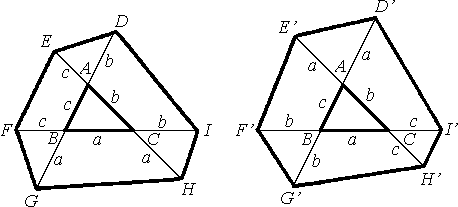Let DABC be given, extend its sides, and construct two hexagons as shown on the right. Compare the areas of the hexagons.

### Solution 1:

Let T(X) denote the area of a figure X, T0 = T(ABC), T1 = T(DEFGHI), and T2 = T(DÆEÆFÆGÆHÆIÆ). We claim that T1 = T2; i. e., the areas of the two hexagons are equal.

Recall that the area of a triangle is given by one-half the product of the lengths of two sides and the sine of the included angle. By side-angle-side, the three ôcornerö triangles of the hexagon on the left, DADE, DBFG, and DCHI, are congruent to DABC. Thus, the area of T1 is

T0 + T(AGH) + T(BDI) + T(CEF) =
 T0 + 1ù2 (a + b)(a + c) sin A + 1ù2 (a + b)(b + c) sin B + 1ù2 (a + c)(b + c) sin C = T0 + 1ù2 [a2 sin A + b2 sin B + c2 sin C + (ab + ac + bc)(sin A + sin B + sin C)],

and the area of T2 is

T(ADÆEÆ) + T(BFÆGÆ) + T(CHÆIÆ) + T(AGÆHÆ) + T(BDÆIÆ) + T(CEÆFÆ) û 2T0 =
 1ù2 [a2 sin A + b2 sin B + c2 sin C + (b + c)2 sin A + (a + c)2 sin B + (a + b)2 sin C] ù 2T0 .
Using the fact that

 T0 = 1ù2 ab sin C = 1ù2 ac sin B = 1ù2 bc sin A,

and in view of the Law of Sines,

(sin A) / a = (sin B) / b = (sin C) / c,

upon expanding the expressions above, it is easy to see that T1 = T2.

### Solution 2:

Note that if two triangles have the same altitude over their bases, then the ratio of their areas equals the ratio of their bases. Thus, in the hexagon on the left, T(ACG) / T0 = (a + c) / c and T(AGH) / T(ACG) = (a + b) / b, so that

 T(AGH)ùùùT0 = T(AGH)ùùùùT(ACG) T(ACG)ùùùùT0 = (a + b)(a + c)ùùùùùùbc .
Similarly,
 T(BDI)ùùùT0 = (a + b)(b + c)ùùùùùùac and T(CEF)ùùùT0 = (a + c)(b + c)ùùùùùùab .
Therefore,
 T1 = T0 + T(AGH) + T(BDI) + T(CEF) = T0 (1 + (a + b)(a + c) / bc + (a + b)(b + c) / ac + (a + c)(b + c) / ab) = T0 (4 + (a + b + c)(a2 + b2 + c2) / abc) .

Since T(ACGÆ) / T0 = (b + c) / c and T(AGÆHÆ) / T(ACGÆ) = (b + c) / b in the hexagon on the right, we find that

 T(AGÆHÆ)ùùùùT0 = T(AGÆHÆ)ùùùùT(ACGÆ) T(ACGÆ)ùùùùT0 = (b + c)2ùùùbc .
Similarly,
 T(BDÆIÆ)ùùùùT0 = (a + c)2ùùùac and T(CEÆFÆ)ùùùùT0 = (a + b)2ùùùab .
Since DADÆEÆ and DAGÆHÆ are similar, T(ADÆEÆ) / T(AGÆHÆ) = a2 / (b + c)2, which combines with T(AGÆHÆ) / T0 = (b + c)2 / bc to yield

 T(ADÆEÆ)ùùùùT0 = T(ADÆEÆ)ùùùùT(AGÆHÆ) T(AGÆHÆ)ùùùùT0 = a2ùbc .
Similarly,
 T(BFÆGÆ)ùùùùT0 = b2ùac and T(CHÆIÆ)ùùùùT0 = c2ùab .
Therefore, T2 = T(ADÆEÆ) + T(BFÆGÆ) + T(CHÆIÆ) + T(AGÆHÆ) + T(BDÆIÆ) + T(CEÆFÆ) û 2T0, which can be expressed in the form

 T0 ( a2ùbc + b2ùac + c2ùab + (b + c)2ùùùùbc + (a + c)2ùùùùac + (a + b)2ùùùùab û 2 ),
which is equal to
 T0(4 + (a + b + c)(a2 + b2 + c2) / abc).
Therefore, T1 = T2.

### Solution 3: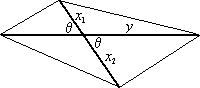One can easily show that if the diagonals of a quadrilateral are of lengths x and y and if they intersect at an angle q, then the area of the quadrilateral is given by (xy sin q) / 2. This generalizes, in degenerate cases, to include the same result for the areas of triangles; for them, x = x1 + x2 = x1 + 0 = x1 in the illustration on the right.

We will use this result to obtain equality of the areas of various triangles and quadrilaterals in the given hexagons.

For instance, we find that T(ABIÆ) = T(ACF) and T(ABHÆ) = T(BCE). Also T(ABHÆIÆ) = T(CEF). Since also T(ABHÆIÆ) = T(ABIÆ) + T(ABHÆ) + T(CHÆIÆ) û T0, we have T(BCE) + T(ACF) + T(CHÆIÆ) = T(CEF) + T0. By similar arguments, T(BCF) + T(ABI) + T(BFÆGÆ) = T(BDI) + T0, and T(ACG) + T(ABH) + T(ADÆEÆ) = T(AGH) + T0.

It can also be seen that T(AGÆHÆ) = T(BCDE), T(BDÆIÆ) = T(ACGF), and T(CEÆFÆ) = T(ABHI). Therefore

 T1 = T(CEF) + T(BDI) + T(AGH) + T0 = [T(CEF) + T0] + [T(BDI) + T0] + [T(AGH) + T0] û 2T0 = [T(BCE) + T(ACF) + T(CHÆIÆ)] + [T(BCD) + T(ABI) + T(BFÆGÆ)] + [T(ACG) + T(ABH) + T(ADÆEÆ)] û 2T0 = [T(BCE) + T(BCD)] + [T(ACF) + T(ACG)] + [T(ABI) + T(ABH)] + T(ADÆEÆ) + T(BFÆGÆ) + T(CHÆIÆ) û 2T0 = T(BCDE) + T(ACGF) + T(ABHI) + T(ADÆEÆ) + T(BFÆGÆ) + T(CHÆIÆ) û 2T0 = T(AGÆHÆ) + T(BDÆIÆ) + T(CEÆFÆ) + T(ADÆEÆ) + T(BFÆGÆ) + T(CHÆIÆ) û 2T0 = T2.

### Comments:

The ratio of the common area of the hexagons to the area of DABC (obtained in Solution 2, in terms of a, b, and c) has some interesting values. It is 13 when DABC is equilateral and 14 when DABC is similar to a 3-4-5 right triangle. Interested readers may wish to pursue an investigation of this ratio further.
IMTS Rounds Index: 1û9 10 11 12 13 14 15 16 17 18 19 20

##Solutions and Comments Round 15

### Problem 1/15

Is it possible to pair off the positive integers 1, 2, 3, ... , 50 in such a manner that the sum of each pair of numbers is a different prime number?

### Solution 1:

Since 49 + 50 = 99 is the largest possible sum and 1 + 2 = 3 is the smallest possible sum, and since only 24 primes pi satisfy 3 ú pi ú 99, such a pairing is not possible.

### Solution 2:

Since 49 + 50 = 99 is the largest possible sum and since there are exactly 25 primes pi less than 99, we must have
 50Si=1 i = 25Si=1 pi
where the piÆs are the 25 lowest primes. However,
 50Si=1 i = 1275 ,   while 25Si=1 pi = 1060 .
Thus, pairing is not possible.

### Solution 3:

Since 49 + 50 = 99 is the largest possible sum and since there are exactly 25 primes pi less than 99, we must use all of them in the pairing. However, for example, we cannot get 2 from any pairing. Therefore such a pairing is not possible.

### Comments:

Alternately, in the spirit of Solution 3, one can observe that 5 cannot be attained since it requires either (2, 3) or (1, 4), but both 1 and 2 must be used to get 3.

More generally, one can show that it is possible to pair off the positive integers 1, 2, 3, 4, ... , 2n û 1, 2n in the desired manner if and only if 1 ú n ú 6 .

### Problem 2/15

Substitute different digits (0, 1, 2, ... , 9) for different letters in the following alphametics to ensure that the corresponding additions are correct. (The two problems are independent of one another.)

 H A R R I E T M A R R I E D + H E R D E N T I S T

 D I A N A A N D S A R A H + A R E R E B E L S

### Solution:

The unique answers are given below:
 3 5 4 4 7 0 9 2 5 4 4 7 0 6 + 3 0 4 6 0 8 9 7 1 9

 3 5 7 0 7 7 0 3 8 7 1 7 6 + 7 1 2 1 2 4 2 9 8

To derive them, one must do a lot of case by case tedious analysis, which we will not reproduce below. In view of the ready availability of speedy home computers, such work is best done via some computer algebra system (like Maple, Mathematica, Macsyma, etc.) or with a computer program (in Pascal, Basic, C, etc.). The key is to devise an algorithm which checks all possible choices for the 10 digits; i. e., all 10! possibilities. While this is a huge number, it shouldnÆt take much more than an hour to complete the task.

Naturally, if one can determine the value of any of the letters, the time can be cut down considerably. It turns out that in both parts of the problem it seems easiest to determine R. Interestingly, while Part (ii) is harder, it is more cumbersome to show that R = 4 in Part (i), than to verify that R = 1 in Part (ii). Once we have R = 4 in Part (i), we can determine the rest of its letters rather easily, even without a computer.

### Problem 3/15

Two pyramids share a seven-sided common base, with vertices labeled as A1, A2, A3, ... , A7, but they have different apexes, B and C . No three of these nine points are colinear. Each of the 14 edges BAi and CAi (i = 1, 2, ... , 7), the 14 diagonals of the common base, and the segment BC are colored either red or blue. Prove that there are three segments among them, all of the same color, that form a triangle.

### Solution 1:

We will assume, without loss of generality, that BC is colored blue, and consider two cases.

In the first case, assume that there are two vertices, Ai and Aj , that are not adjacent to one another, with both of the edges, BAi and BAj , colored blue. Then, to avoid creating a monochromatic triangle (one, whose three sides are of the same color), we must color CAi red (in view of DBCAi), and CAj must also be red (in view of DBCAj). However, the coloring of diagonal AiAj will make either DBAiAj, or DCAiAj monochromatic. Hence this case leads to a monochromatic triangle.

In the second case, we may assume that there are five adjacent vertices, say A3, A4, A5, A6, A7 such that the edges BA3, BA4, BA5, BA6, BA7 are all blue. Again we will try to avoid creating a monochromatic triangle by careful coloring of the diagonals of the heptagon. To that end, A3A7 must be blue (in view of DBA3A7), A4A6 must be blue (in view of DBA4A6), A3A5 must be blue (in view of DBA3A5), and A5A7 must be blue (in view of DBA5A7). However, since all three sides of DA3A5A7 are blue, this case also leads to a monochromatic triangle.

### Solution 2:

Assume there is no triangle with three sides of the same color. Suppose, with no loss of generality, that BC is colored blue. Then, for each pair of segments BAi and CAi, at least one segment must be red. Therefore there are at least seven red edges between the apexes and the base, so there must be at least four at one apex, call it B . If there were five red edges emanating from an apex, at least three of the endpoints at the base would be the vertices of a triangle in the base, so we can assume that there are four red and three blue edges between B and the base. Designate the three base vertices that have a blue edge to B as Ai, Aj and Ak . Then CAi, CAj and CAk are all red. Since at least two of Ai, Aj and Ak are joined by a diagonal, that diagonal cannot be r,ed (it would create a red triaugle with B) and cannot be blue (it would create a red triangle with B) and cannot be blue (it would create a blue triangle with C). This contradiction proves that there must be a triangle having all three sides the same color.

### Comments:

This problem, like many graph theoretic problems involving a two-coloring of the edges, is related to a fascinating area of mathematics known as Ramsey Theory. Most graph theory books have some introductory material on Ramsey theory.

To follow the solutions given above, the reader is advised to make his/her own sketches of the different configurations presented.

### Problem 4/15

Suppose that for positive integers a, b, c and x, y, z, the equations a2 + b = c2 and x2 + y2 = z2 are satisfied. Prove that

(a + x)2 + (b + y)2 ú (c + z)2,

and determine when equality holds.

### Solution 1: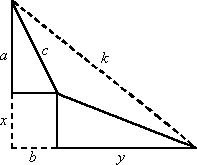In the figure on the right, in view of the Triangle Inequality, k ú c + z . Since k2 = (a + x)2 + (b + y)2 , this is equivalent to (a + x)2 + (b + y)2 ú (c + z)2 . Equality holds if and only if k = c + z , i. e., if and only if the right triangles with sides a, b, c and x, y, z are similar, i. e., if and only if a/z = b/y = c/z .

### Solution 2: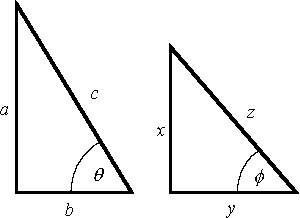Since (a/c)(x/z) + (b/c)(y/z) = sin q sin f + cos q cos f = cos(q û f) ú 1; and it follows that ax + by ú cz . Therefore, (a + x)2 + (b + y)2 = (a2 + b2) + (x2 + y2) + 2(ax + by) ú c2 + z2 + 2cz = (c + z)2. Equality holds if and only if q = f . In that case, the triangles are similar; i. e., a/x = b/y = c/z .

### Solution 3:

For positive a, b, c, x, y, z, with a2 + b2 = c2 and x2 + y2 = z2, 0 ú (ay û bx)2 2aybx ú a2y2 + b2x2 (ax + by)2 = a2x2 + 2axby + b2y2 ú a2x2 + a2y2 + b2x2 + b2y2 = (a2 + b2)(x2 + y2) = c2z2 ax + by ú cz (a + x)2 + 2(ax + by) + (x2 + y2) ú c2 + 2cz + z2 = (c + z)2 . Equality holds if and only if ay û bx = 0, in which case a/x = b/y , implying that c2/z2 = (a2 + b2) / (x2 + y2) = ((b2x2 / y2) + b2) / (x2 + y2) = b2/y2 ; i. e., c/z = b/y .

### Solution 4:

Let u = (a, b) and v = (x, y) . Then c = Í(a2 + b2) = ||u|| and z = Í(x2 + y2) = ||v|| . In viev of the Triangle Inequality for vectors in the plane it follows that Í((a + x)2 + (b + y)2) = ||u + v|| ú ||u|| + ||v|| = c + z , which is equivalent to the desired inequality. Equality occurs if and only if u = kv for some real constant k , i. e., if and only if a = kx and b = ky . In that case, for positive k , c = ||u|| = ||kv|| = k||v|| = kz .

### Comments:

One can also use as an alternate starting point the Cayley-Schwarz Inequality, (a2 + b2)(x2 + y2) (ax + by)2 , which follows from the fact that (a2 + b2)(x2 + y2) = (ax + by)(ay û bx)2 .

More generally, one can also show that if ai and bi are positive real numbers for 1 ú i ú n , where n is a positive integer greater than 2, and

 if nSi=1 ai2 = x2 and nSi=1 bi2 = y2 , then nSi=1 (ai + bi)2 ú (x + y)2 ,
with equality if and only if a1/b1 = a2/b2 = ... = an/bn .

In the more restricted setting of the present problem, the result can also be derived by first showing that if (a, b, c) and (x, y, z) are primitive Pythagorian triples (i. e., the greatest common divisor of a, b, c is 1, and the same is true for x, y, z), then (c + z)2 û (a + x)2 û (b + y)2 is the square of a non-negative integer.

### Problem 5/15

Let C1 and C2 be two circles intersecting at the points A and B , and let C0 be a circle through A , with center at B . Determine, with proof, conditios under which the common chord of C0 and C1 is tangent to C2?

### Solution 1:

Let O denote the center of circle C1 , P denote the center of circle C2 , and Q denote the point of intersection of circles C1 and C0 , other than A . We claim that AQ is tangent to C2 at A if and only if the circles C1 and C2 have equal radii. To this end, let r1 = OA = OB be the radius of C1 and r2 = PA = PB be the radius C2 .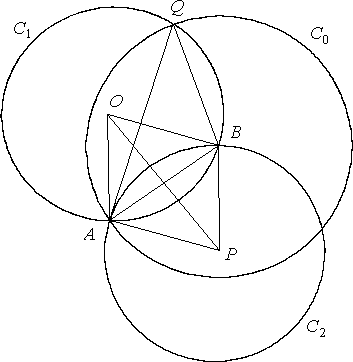Note that the segment connecting the centers of two intersecting circles is the perpendicular bisector of their common chord. Therefore, AB ^ OP and AQ ^ OB. Now assume that AQ is tangent to C2 at A . Then AQ ^ AP . Therefore, AP is parallel to OB and so ðAPO = ðBOP . Moreover, ðAPO = ðBPO, so ðBOP = ðBPO . Therefore, DBOP is isosceles with equal sides OB and PB . So r1 = OB = PB = r2 .

Conversely, assume that r1 = r2 . Then AOBP is a rhombus so that AP is parallel to OB . Since AQ ^ OB , it follows that AQ ^ AP . Hence AQ is tangent to C2 at A .

### Solution 2:

Let a = ðBAQ = ðAQB and b = ðBAP = ðABP . Note that the sine of any angle in a triangle is equal to the ratio of the length of the side opposite the angle to the length of the diameter of the circumscribed circle. Applying this result to the angle a = ðAQB in DBAQ (which C1 circumscribes), we have sin a = AB/2r1 . Also, applying the Law of Cosines in DABP , we have

 cos b = r22 + AB2 û r22ùùùùùù2r2AB = ABùù2r2 .

Now, AQ is tangent to C2 at A a + b = 90░ sin a = cos b AB/2r1 = AB/2r2 r1 = r2 .

IMTS Rounds Index: 1û9 10 11 12 13 14 15 16 17 18 19 20

##Problems Round 16

### Problem 1/16

Prove that if a + b + c = 0 , then a3 + b3 + c3 = 3abc .

### Problem 2/16

For a positive integer n, let P(n) be the product of the nonzero base 10 digits of n . Call n ôprodigitiousö if P(n) divides n . Show that one can not have a sequence of fourteen consecutive positive integers that are all prodigitious.

### Problem 3/16

Disks numbered 1 through n are placed in a row of squares, with one square left empty. A move consists of picking up one of the disks and moving it into the empty square, with the aim to rearrange the disks in the smallest number of moves so that disk 1 is in square 1, disk 2 is in square 2, and so on until disk n is in square n and the last square is empty. For example, if the initial arrangement is

 3 2 1 6 5 4 9 8 7 12 11 10

then it takes at least 14 moves; i. e., we could move the disks into the empty square in the following order: 7, 10, 3, 1, 3, 6, 4, 6, 9, 8, 9, 12, 11, 12.

What initial arrangement requires the largest number of moves if n = 1995? Specify the number of moves required.

### Problem 4/16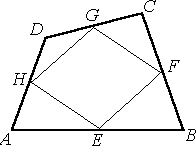Let ABCD be an arbitrary convex quadrilateral, with E, F, G, H the midpoints of its sides, as shown in the figure on the right. Prove that one can piece together triangles AEH, BEF, CFG, DGH to form a parallelogram congruent to parallelogram EFGH .

### Problem 5/16

An equiangular octagon ABCDEFGH has sides of length 2, 2Í2, 4, 4Í2, 6, 7, 7, 8. Given that AB = 8 , find the length of EF .
IMTS Rounds Index: 1û9 10 11 12 13 14 15 16 17 18 19 20

##Problems Round 17

### Problem 1/17

The 154-digit number, 19202122...939495, was obtained by listing the integers from 19 to 95 in succession. We are to remove 95 of its digits, so that the resulting number is as large as possible. What are the first 19 digits of this 59-digit number?

### Problem 2/17

Find all pairs of positive integers (m,n) for which m2 û n2 = 1995.

### Problem 3/17

Show that it is possible to arrange in the plane 8 points so that no 5 of them will be the vertices of a convex pentagon. (A polygon is convex if all of its interior angles are less than or equal to 180░.)

### Problem 4/17

A man is 6 years older than his wife. He noticed 4 years ago that he has been married to her exactly half of his life. How old will he be on their 50th anniversary if in 10 years she will have spent two-thirds of her life married to him?

### Problem 5/17

What is the minimum number of 3 Î 5 rectangles that will cover a 26 Î 26 square? The rectangles may overlap each other and/or the edges of the square. You should demonstrate your conclusion with a sketch of the covering.
IMTS Rounds Index: 1û9 10 11 12 13 14 15 16 17 18 19 20

##Problems Round 18

### Problem 1/18

Determine the minimum length of the interval [a, b] such that a ú x + y ú b for all real numbers x y 0 for which 19 x + 95 y = 1995.

### Problem 2/18

For a positive integer n 2, let P(n) denote the product of the positive integer divisors (including 1 and n) of n. Find the smallest n for which P(n) = n10.

### Problem 3/18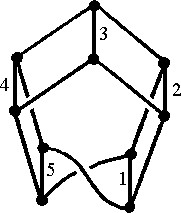The graph shown on the right has 10 vertices, 15 edges, and each vertex is of order 3 (i. e., at each vertex 3 edges meet). Some of the edges are labeled 1, 2, 3, 4, 5 as shown. Prove that it is possible to label the remaining edges 6, 7, 8, ... , 15 so that at each vertex the sum of the labels on the edges meeting at that vertex is the same.

### Problem 4/18

Let a, b, c, d be distinct real numbers such that

a + b + c + d = 3   and   a2 + b2 + c2 + d2 = 45.

Find the value of the expression

 a5ùùùùùùùùùù(a û b)(a û c)(a û d) + b5ùùùùùùùùùù(b û a)(b û c)(b û d) + c5ùùùùùùùùùù(c û a)(c û b)(c û d) + d5ùùùùùùùùùù(d û a)(d û b)(d û c) .

### Problem 5/18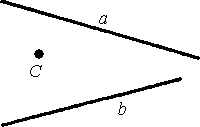Let a and b be two lines in the plane, and let C be a point as shown in the figure on the right. Using only a compass and an unmarked straight edge, construct an isosceles right triangle ABC, so that A is on line a, B is on line b, and AB is the hypotenuse of DABC.

IMTS Rounds Index: 1û9 10 11 12 13 14 15 16 17 18 19 20

##Problems Round 19

### Problem 1/19

It is possible to replace each of the ▒ signs below by either û or + so that

▒ 1 ▒ 2 ▒ 3 ▒ 4 ▒ ... ▒ 96 = 1996.

At most how many of the ▒ signs can be replaced by a + sign?

### Problem 2/19

We say (a, b, c) is a primitive Heronian triple if a, b, and c are positive integers with no common factors (other than 1), and if the area of the triangle whose sides measure a, b, and c is also an integer. Prove that if a = 96, then b and c must both be odd.

### Problem 3/19

 5 5 5 2 1 3 3 4 6 4 4 2 1 1 5 2 6 3 3 2 1 6 0 3 3 0 5 5 0 0 0 6 3 2 1 6 0 0 4 2 0 3 6 4 6 2 6 5 2 1 1 4 4 4 1 5
The numbers in the 7Î8 rectangle shown on the right were obtained by putting together the 28 distinct dominoes of a standard set, recording the number of dots, ranging from 0 to 6 on each side of the dominoes, and then erasing the boundaries among them. Determine the original boundaries among the dominoes. (Note: Each domino consists of two adjoint squares, referred to as its sides.)

### Problem 4/19

Suppose that f satisfies the functional equation
 2 f (x) + 3 f ( 2 x + 29ùùùùx û 2 ) = 100 x + 80.
Find f (3).

### Problem 5/19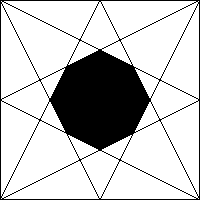In the figure on the right, determine the area of the shaded octagon as a fraction of the area of the square, where the boundaries of the octagon are lines drawn from the vertices of the square to the midpoints of the opposite sides.

IMTS Rounds Index: 1û9 10 11 12 13 14 15 16 17 18 19 20
Prepared by Vladimir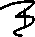ensa. Last updated on 2nd August, 1998.